MORE IN Mechanics of Solids
GTU Civil Engineering (Semester 3)
Mechanics of Solids
May 2015
Total marks: --
Total time: --
INSTRUCTIONS
(1) Assume appropriate data and state your reasons
(2) Marks are given to the right of every question
(3) Draw neat diagrams wherever necessary

1 (a) Explain following terms (i) Rigid body, (ii) Deformable body (iii) Elastic body.
3 M
1 (b) State "Hooks Law". Derive formula to determine change in length (δL) for the uniform, homogeneous axially loaded member of length (L), c/s area (A) and modulus of elasticity (E), subjected to axial tensile force (P).
4 M
1 (c) A stepped circular bar ABC is axially loaded as shown in fig. (i), is in equilibrium. The diameter of part AB is 50 mm throughout its length, whereas diameter part BC is uniform decreasing from 40 mm at B to 30 mm at C. Determine (i) magnitude of unknown force "P" (ii) stress in part AB and (iii) change in length of part BC. Take modulus of elasticity =2×105N/mm2.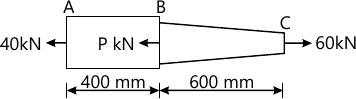7 M

2 (a) Derive relation between Rate of loading, Shear force and bending moment at a section in a beam subjected to general loading. Also derive condition for maximum BM in terms of SF.
7 M
Answer any one question from Q2 (b) & Q2 (c)
2 (b) Find magnitude and inclination with +X-axis of resultant of force system shown in fig (ii). Identify type of force system.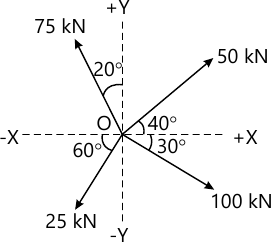7 M
2 (c) A cylindrical roller weighing 1000 N is resting between two smooth surfaces inclined at 60° and 30° with horizontal as shown in fig. (iii). Draw free body diagram and determine reactions at contact points A and B.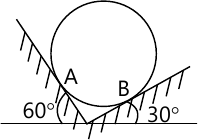7 M

Answer any two question from Q3 (a), (b) & Q3 (c), (d)
3 (a) Calculate shear force and bending moment at salient points for the beam shown in fig. (iv). Also plot neat shear force and bending moment diagrams indicating values at above points. Locate point of contraflexure from support B.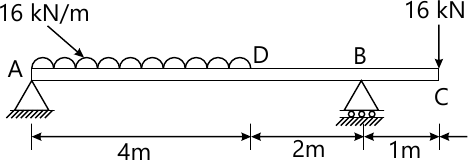7 M
3 (b) A reinforced concrete column 500 mm×500 mm in section is reinforced with four steel bars of 25 mm diameter, one in each corner. The column is carrying an axial load of 1000 kN. Find the stresses in concrete and steel bars. Take E for steel = 210 GPa and E for concrete = 14 Gpa.
7 M
3 (c) Explain (i) Angle of limiting friction (ϕlim), (ii) Angle of repose (λ), and (iii) Coefficient of friction (μ) . Also prove that ϕlim and λ are numerically same.
7 M
3 (d) Determine the location of centroid of plane lamina shown in fig. (v) with respect to point O.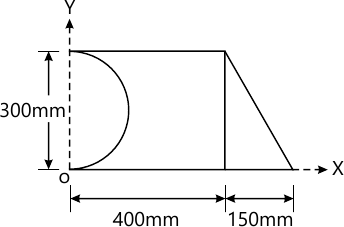7 M

Answer any two question from Q4 (a), (b) & Q4 (c), (b)
4 (a) Two blocks are connected by a horizontal link AB are supported on two rough planes as shown in fig. (vi). The coefficient of friction for block A is 0.4 and angle of friction for block B is Ï•= 15° What is the smallest weight WA of the block A for which the equilibrium of the system can exist?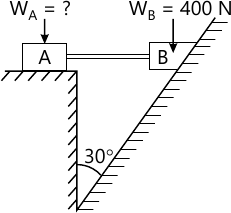7 M
4 (b) State Parallel axis theorem and perpendicular axis theorem. Derive formula for moment of Inertia of a rectangular section about horizontal centroidal axis from first fundamental. Using parallel axis theorem derive formula for moment of inertia about base of the section.
7 M
4 (c) A steel circular bar of 16 mm diameter is placed inside a copper tube, having internal diameter of 20 mm and thickness of 2.5 mm as shown in fig. (vii). Both the ends are rigidly fixed and initially stress free. If the temperature of assembly is increased by 50°C, compute magnitude and nature of stresses produced in each material. Take modulus of elasticity of steel and copper as 200 GPa and 100 GPa respectively. Take coefficient of thermal expansion (per °C) for steel and copper as 12×10-6 and 18×10-6 respectively.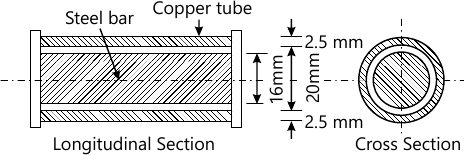7 M
4 (d) A rectangular block is 205 mm long, 100 mm wide and 80 mm thick. It is subjected to a tensile load of 200 kN, compressive load of 300 kN and tensile load of 250 kN along its length, width and thickness respectively. Find the change in volume of the block. Also calculate shear modulus for the block material. Take E = 210 GPa and Poisson's ratio ν =0.25.
7 M

Answer any two question from Q5(a), (b) & Q5 (c), (d)
5 (a) Draw bending stress distribution diagram across the cross section of a "T" beam, having flange 150×20 mm and web 20×250 mm, carrying pure hogging bending moment of 30 kNm at the section.
7 M
5 (b) Write the assumptions for finding out shear stress in a circular shaft, subjected to torsion. Prove that τ/R = Cθ/L with usual notations for circular shaft.
7 M
5 (b) A machine component is subjected to the stresses as shown in fig. (viii). Find the normal and shearing stresses on the section AB inclined at an angle of 60° with horizontal (x-x axis). Also find the resultant stress on the section.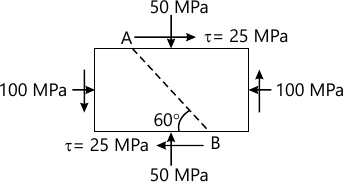7 M
5 (c) Draw shear stress distribution diagram across the cross section of a "T" beam, having flange 150×20 mm and web 20×250 mm, carrying pure shear force of 50 kN at the section.
7 M

More question papers from Mechanics of Solids